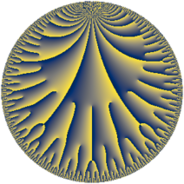# Properties

 Label 75.22.bLevel $75$ Weight $22$ Character orbit 75.b Rep. character $\chi_{75}(49,\cdot)$ Character field $\Q$ Dimension $64$ Newform subspaces $10$ Sturm bound $220$ Trace bound $4$

# Related objects

## Defining parameters

 Level: $$N$$ $$=$$ $$75 = 3 \cdot 5^{2}$$ Weight: $$k$$ $$=$$ $$22$$ Character orbit: $$[\chi]$$ $$=$$ 75.b (of order $$2$$ and degree $$1$$) Character conductor: $$\operatorname{cond}(\chi)$$ $$=$$ $$5$$ Character field: $$\Q$$ Newform subspaces: $$10$$ Sturm bound: $$220$$ Trace bound: $$4$$ Distinguishing $$T_p$$: $$2$$

## Dimensions

The following table gives the dimensions of various subspaces of $$M_{22}(75, [\chi])$$.

Total New Old
Modular forms 216 64 152
Cusp forms 204 64 140
Eisenstein series 12 0 12

## Trace form

 $$64 q - 66781932 q^{4} + 31414068 q^{6} - 223154201664 q^{9} + O(q^{10})$$ $$64 q - 66781932 q^{4} + 31414068 q^{6} - 223154201664 q^{9} - 119566210064 q^{11} + 2024808384492 q^{14} + 83961995679540 q^{16} - 180551235585468 q^{19} + 93267553251996 q^{21} + 306221028356196 q^{24} - 1498611616977572 q^{26} - 4502526146038856 q^{29} + 12082507390298404 q^{31} + 92844941666377336 q^{34} + 232854198766242732 q^{36} - 90183258672430932 q^{39} + 406214591801409464 q^{41} + 407746489839931144 q^{44} + 540801265580066184 q^{46} - 7800922240899827740 q^{49} + 2097031371496605072 q^{51} - 109534082274353268 q^{54} + 7927226749838652000 q^{56} + 17465146979255103248 q^{59} + 37182020949747667260 q^{61} - 184180107580762691668 q^{64} + 66861170132144054544 q^{66} + 2177675206221816792 q^{69} + 197896027049926832288 q^{71} - 150289592695649953448 q^{74} + 757538041256819757152 q^{76} + 5476181972666438000 q^{79} + 778090589379643443264 q^{81} - 565675864178348002392 q^{84} + 1194199728718529165836 q^{86} + 69369005718939001992 q^{89} + 2528553323851077528404 q^{91} - 4364562020368042786472 q^{94} + 188022191429571626916 q^{96} + 416901596137844411664 q^{99} + O(q^{100})$$

## Decomposition of $$S_{22}^{\mathrm{new}}(75, [\chi])$$ into newform subspaces

Label Dim $A$ Field CM Traces $q$-expansion
$a_{2}$ $a_{3}$ $a_{5}$ $a_{7}$
75.22.b.a $2$ $209.608$ $$\Q(\sqrt{-1})$$ None $$0$$ $$0$$ $$0$$ $$0$$ $$q+2844iq^{2}-3^{10}iq^{3}-5991184q^{4}+\cdots$$
75.22.b.b $2$ $209.608$ $$\Q(\sqrt{-1})$$ None $$0$$ $$0$$ $$0$$ $$0$$ $$q+12^{3}iq^{2}+3^{10}iq^{3}-888832q^{4}+\cdots$$
75.22.b.c $2$ $209.608$ $$\Q(\sqrt{-1})$$ None $$0$$ $$0$$ $$0$$ $$0$$ $$q+544iq^{2}-3^{10}iq^{3}+1801216q^{4}+\cdots$$
75.22.b.d $4$ $209.608$ $$\Q(i, \sqrt{649})$$ None $$0$$ $$0$$ $$0$$ $$0$$ $$q+(333\beta _{1}+\beta _{2})q^{2}-3^{10}\beta _{1}q^{3}+(-589618+\cdots)q^{4}+\cdots$$
75.22.b.e $6$ $209.608$ $$\mathbb{Q}[x]/(x^{6} + \cdots)$$ None $$0$$ $$0$$ $$0$$ $$0$$ $$q+(\beta _{1}+268\beta _{2})q^{2}-3^{10}\beta _{2}q^{3}+(-2238318+\cdots)q^{4}+\cdots$$
75.22.b.f $6$ $209.608$ $$\mathbb{Q}[x]/(x^{6} + \cdots)$$ None $$0$$ $$0$$ $$0$$ $$0$$ $$q+(-234\beta _{1}+\beta _{2})q^{2}-3^{10}\beta _{1}q^{3}+\cdots$$
75.22.b.g $6$ $209.608$ $$\mathbb{Q}[x]/(x^{6} + \cdots)$$ None $$0$$ $$0$$ $$0$$ $$0$$ $$q+(767\beta _{1}+\beta _{4})q^{2}+3^{10}\beta _{1}q^{3}+(-421908+\cdots)q^{4}+\cdots$$
75.22.b.h $8$ $209.608$ $$\mathbb{Q}[x]/(x^{8} + \cdots)$$ None $$0$$ $$0$$ $$0$$ $$0$$ $$q+(\beta _{1}+224\beta _{5})q^{2}-3^{10}\beta _{5}q^{3}+(290854+\cdots)q^{4}+\cdots$$
75.22.b.i $12$ $209.608$ $$\mathbb{Q}[x]/(x^{12} + \cdots)$$ None $$0$$ $$0$$ $$0$$ $$0$$ $$q+(-15\beta _{5}+\beta _{6})q^{2}-3^{10}\beta _{5}q^{3}+(-731822+\cdots)q^{4}+\cdots$$
75.22.b.j $16$ $209.608$ $$\mathbb{Q}[x]/(x^{16} + \cdots)$$ None $$0$$ $$0$$ $$0$$ $$0$$ $$q+(83\beta _{8}+\beta _{9})q^{2}+3^{10}\beta _{8}q^{3}+(-1335201+\cdots)q^{4}+\cdots$$

## Decomposition of $$S_{22}^{\mathrm{old}}(75, [\chi])$$ into lower level spaces

$$S_{22}^{\mathrm{old}}(75, [\chi]) \cong$$ $$S_{22}^{\mathrm{new}}(5, [\chi])$$$$^{\oplus 4}$$$$\oplus$$$$S_{22}^{\mathrm{new}}(15, [\chi])$$$$^{\oplus 2}$$$$\oplus$$$$S_{22}^{\mathrm{new}}(25, [\chi])$$$$^{\oplus 2}$$